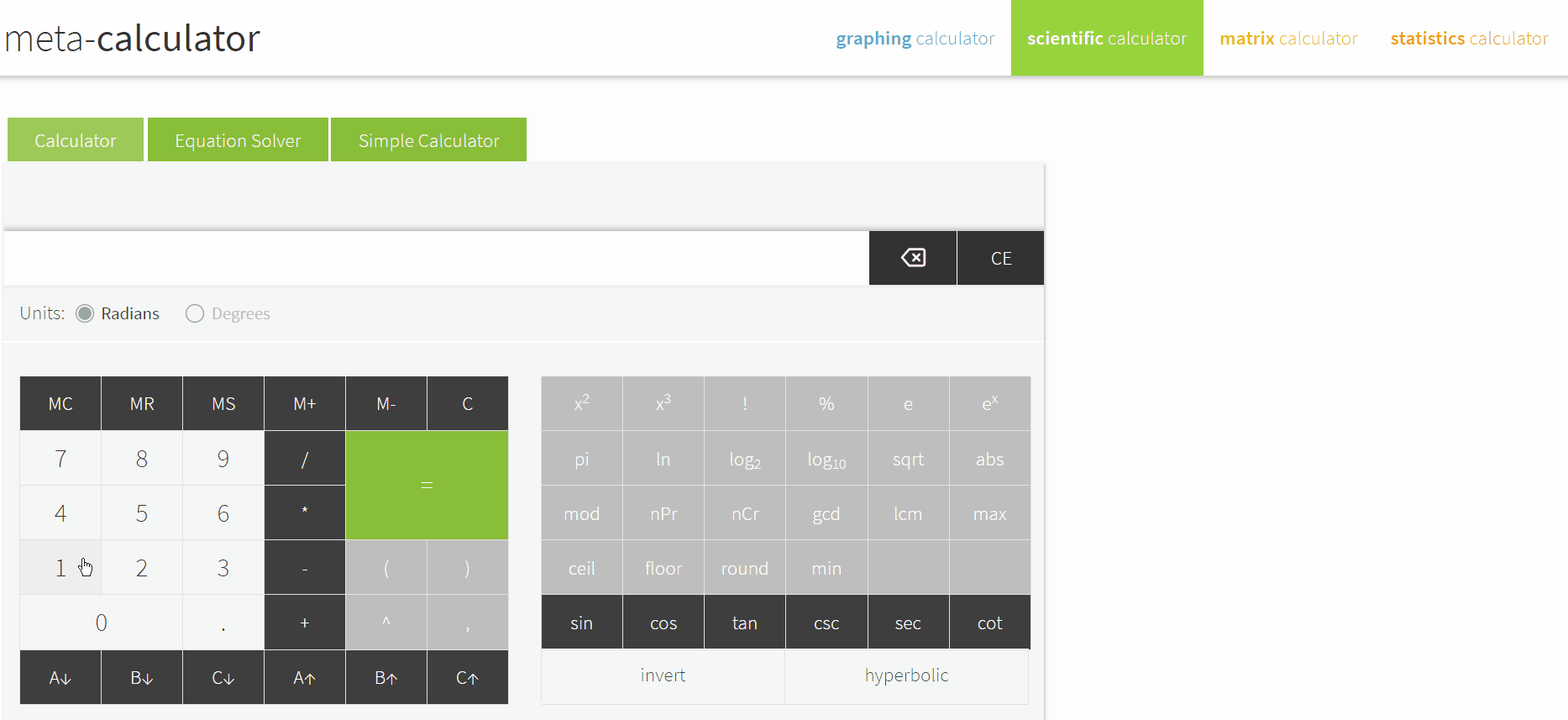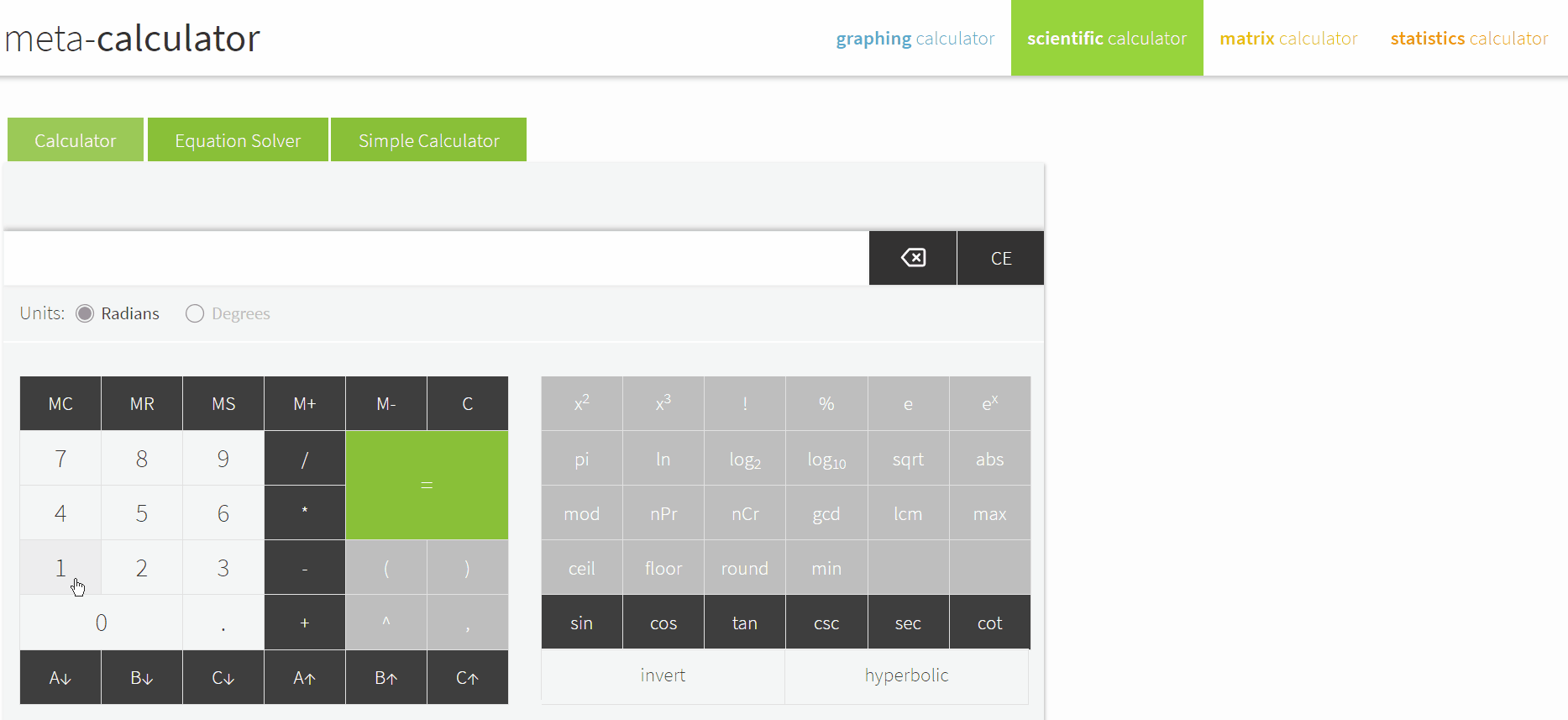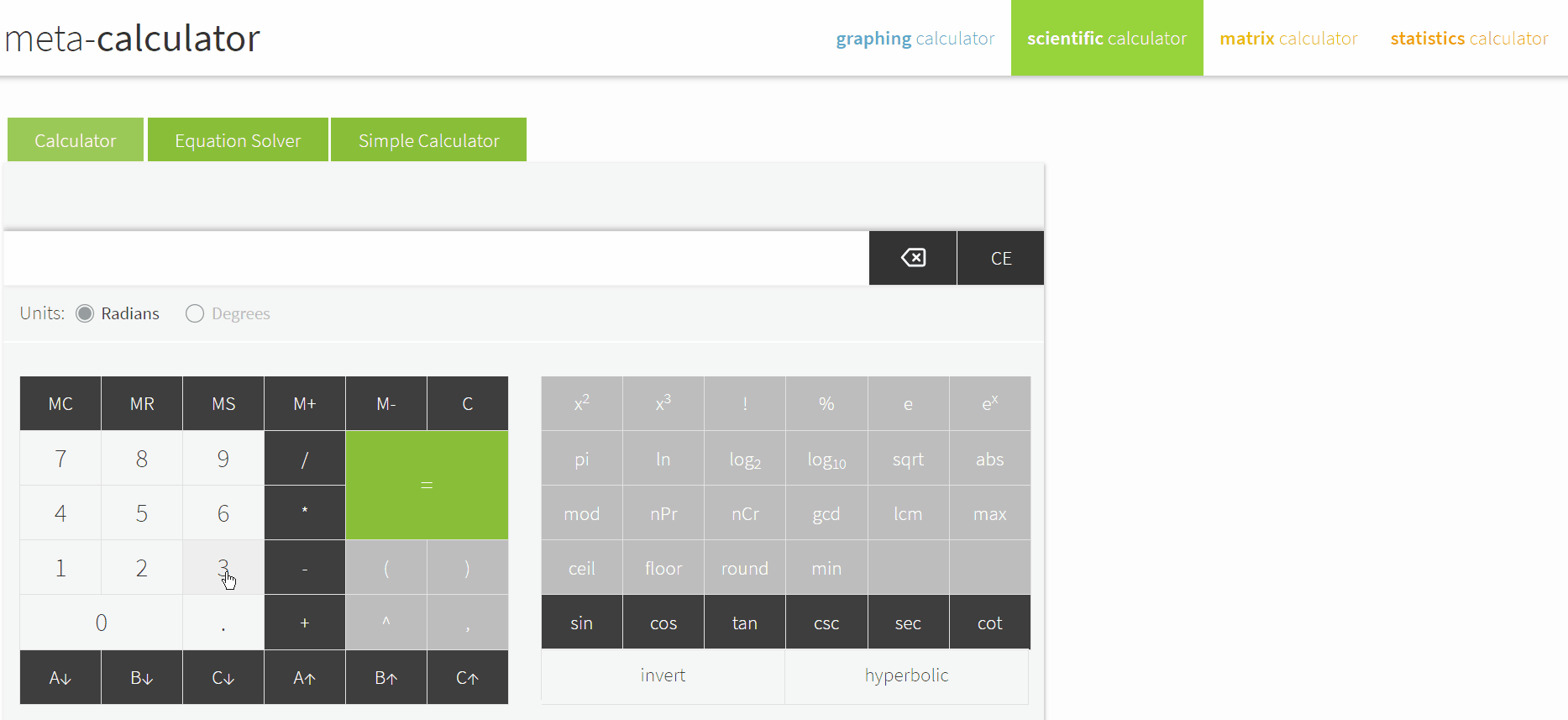# How To Use Log On Scientific Calculator

Logarithms are easy to understand. They are functions that help you determine what exponents must be multiplied into a specific number.

As you move on to learn advanced math, you will need to use scientific calculators to calculate log values quickly.

These calculators are very different from basic and business calculators and come loaded with functions that help solve physics, engineering, and trigonometric problems.

Thankfully, working with logarithmic functions on a scientific calculator is easy – after you learn to do it right.

# How To Use Log On Scientific Calculator

## Calculating Common Logarithms with A Scientific Calculator

### Step #1: Enter the Number

Turn your calculator on, and type in the number you want to calculate the common logarithm (log base 10) of.

Let’s use “1000” as an example.

### Step #2: Press the Log Button

To apply the log function on the number, click on the log button on the calculator.

A number will appear on the screen immediately after you click the log button. The figure represents the number of times you have to multiply 10 with itself to get the original number.### Step #3: Verify the Answer (Optional)

You can go ahead and check your work to ensure you got all the steps right. In our example, “3” appears as the result. Multiplying 10 with itself thrice gives us 1000, which means the answer is correct.

While there is no antilog calculator in scientific calculators, you can use the result of the logarithmic calculation as an exponent to 10 to find the antilog of any log.

## Calculating Natural Logarithms With A Scientific Calculator

Natural logarithms are the logarithms that have base “e,” and on most scientific calculators, these are represented by the “ln” button.

To use the ln key to find a logarithm with base e:

### Step #1: Enter the Number

Enter the number you want to find the natural logarithm of. Let’s say it’s 10.

### Step #2: Find and Press the “ln” Button

You must then click on the “ln” button on the calculator. A result will appear immediately.

The result is the number to which the irrational base “e” must be raised to obtain the argument.## Calculating Log with Other Bases on A Scientific Calculator

Most scientific calculators only come with the log and ln buttons. There is no button for finding logarithms with other bases.

To work out a logarithm with another base, you must use the change of base formula, which is:

a=a b

So, if you want to find the value of 3, you must calculate 3 2 .

To do this on your scientific calculator:

### Step #1: Enter the Numerator

To do this, type in the argument of your logarithm into the calculator. In our example, the argument is 3.

Then, hit the “ln” button on the calculator. But don’t hit the “=” just yet.

### Step #2: Enter the Denominator

After entering the numerator, find and click on the “divide” button on the calculator. You must then feed the denominator into the calculator.

To do that, hit the “ln” button first. The natural log function will appear with a single parenthesis. You must then type in the argument of the logarithm, which is 2 in this example.

To trigger the calculation, enter an ending parenthesis in the calculator.

The result will appear on the screen, indicating the value of 3 .## Conclusion

As you can see, calculating the log of any number is quite easy using a scientific calculator. After you use it a few times, you will get the hang of where the buttons are and will be able to find the values quickly.

We have various comprehensive calculators that you can use online for free. You can choose from t-test calculator, graphing, matrix, the standard deviation to statistics, and scientific calculators. Check it here